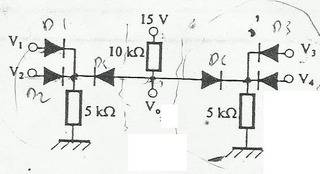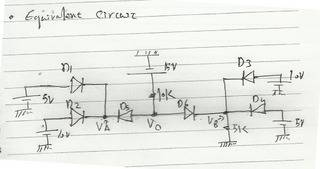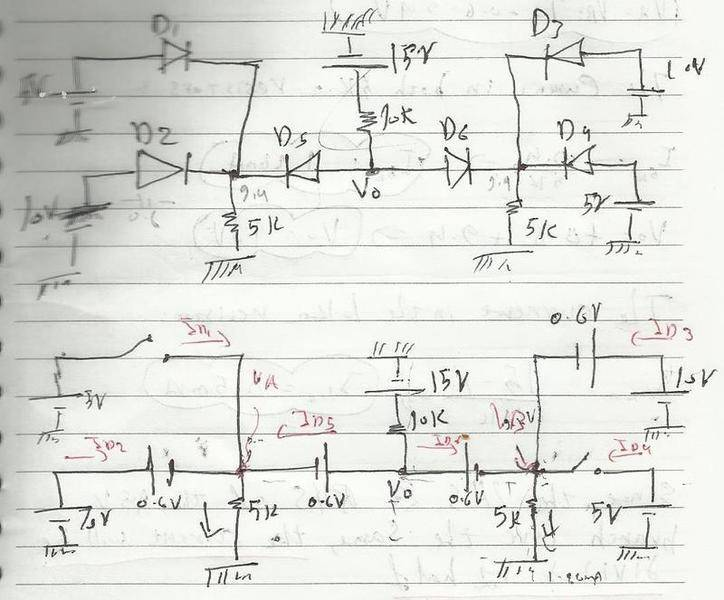# Multiple Diodes Problem

## Homework Statement

If V(1)=10 V and V(0)=5 V, and the voltage threshold for all diodes is 0.6 V. Determine the current through every diode and the output voltage Vo if V1=V4= 5V and V2=V3=10 V.Basic KCL & KVL.

## The Attempt at a Solution

I know that the basic idea to determine whether the diode is on or off is by assuming one state and then confirm it. The thing is I could do that with two or three diodes at most. but I know another to determine its state which is done by determining the voltages on both the anode and the cathode separately. And that I what I was going, but I couldn't determine some voltages like VA and VB in the following figure:Any help?

gneill
Mentor
Certain diodes being turned on will dominate things. Once a diode is on it will effectively "clamp" the potential to one diode-drop from the source that's driving that current. So look for the highest potential sources first and assume their diodes are on. See what that would imply for the others (look for contradictions).

In your equivalent circuit diagram you seem to have "lost" a 5k resistor, and the 10k resistor seems to have become a 1k resistor. You also left out the 5V source for Vo.

NascentOxygen
Staff Emeritus
You also left out the 5V source for Vo.
There is no 5V source at Vo. Vo is our output.

Vo is different from V(0).V(0) = 5V is just telling us that for this gate, LOGIC LOW is approx 5V

gneill
Mentor
There is no 5V source at Vo. Vo is our output.

Vo is different from V(0).V(0) = 5V is just telling us that for this gate, LOGIC LOW is approx 5V
Ah. I should have spotted that. Thanks.

My suggested approach still applies though.

Sorry for the "typo" ^^"

Anyway, this is what I've done:http://s32.postimg.org/da0hvrf5h/004.jpg

Am I doing OK?

gneill
Mentor
Looking good.

Thanks. I really appreciate your help. :)